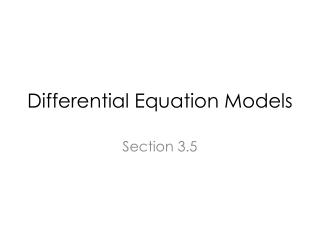DownloadDownload PresentationDifferential Equation Models

# Differential Equation Models

Download Presentation## Differential Equation Models

- - - - - - - - - - - - - - - - - - - - - - - - - - - E N D - - - - - - - - - - - - - - - - - - - - - - - - - - -
##### Presentation Transcript

1. Differential Equation Models Section 3.5

2. Impulse Response of an LTI System

3. H(s) H(s) is the the Laplace transform of h(t) With s=jω, H(jω) is the Fourier transform of h(t) Cover Laplace transform in chapter 7 and Fourier Transform in chapter 5. H(s) can also be understood using the differential equation approach.

4. Complex Exponential

5. RL Circuit Let y(t)=i(t) and x(t)=v(t) Differential Equation & ES 220

6. nth order Differential Equation • If you use more inductors/capacitors, you will get an nth order linear differential equation with constant coefficients

7. Solution of Differential Equations • Find the natural response • Find the force Response • Coefficient Evaluation

8. Determine the Natural Response • Let L=1H, R=2Ω & =2 • (0≤t) • Condition: y(t=0)=4 • Assume yc(t)=Cest • Substitute yc(t) into • What do you get? 0, since we are looking for the natural response.

9. Natural Response (Cont.) • Substitute yc(t) into Assume yc(t)=Cest

10. Nth Order System Assume yc(t)=Cest (characteristic equation) (no repeated roots)

11. Stability ↔Root Locations (unstable) Stable (marginally stable)

12. The Force Response • Determine the form of force solution from x(t) Solve for the unknown coefficients Pi by substituting yp(t) into

13. Finding The Forced Solution

14. Finding the General Solution (initial condition)

15. Nth order LTI system • If there are more inductors and capacitors in the circuit,

16. Transfer Function (Transfer function)

17. Summary (p. 125)

18. Summary (p. 129)